# What is Work?

• Work is the process of moving an object by applying a force

• The object must move for work to be done

• The force must cause the movement

• Work is a scalar quantity

• Units are Joules

# Work in One Dimension

• Only the force in the direction of the displacement contributes to the work done# Non-Constant Forces

• Work done is the area under the force vs. displacement graph# Hooke's Law

• The more you stretch or compress a string, the greater the force of the spring

• The spring's force is opposite the direction of its displacement from equilibrium

• Model this as a linear relationship, where the force applied by the spring is equal to a constant (the spring constant) multiplied by the spring's displacement from its equilibrium (rest) position

•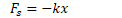• Slope of the graph gives you the spring constant, k (in N/m)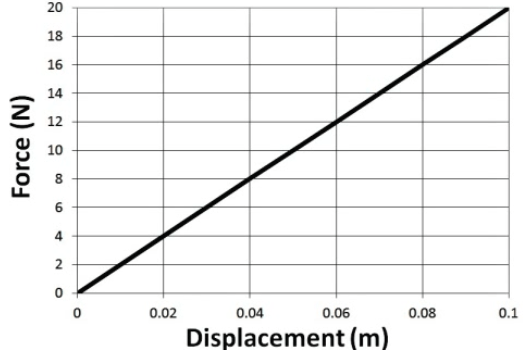# Work Done in Compressing a Spring

• A spring obeys Hooke's Law. How much work is done in compressing the spring from equilibrium to some point x?# Work Done in Compressing a Non-Linear Spring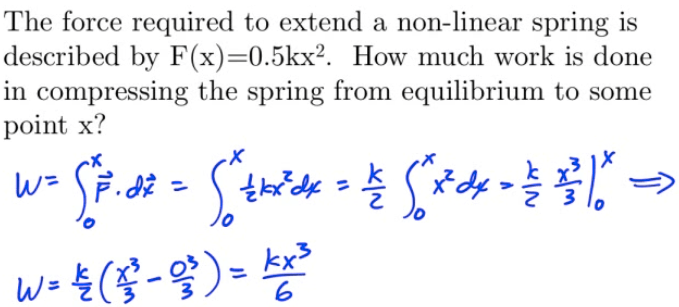# Work in Multiple Dimensions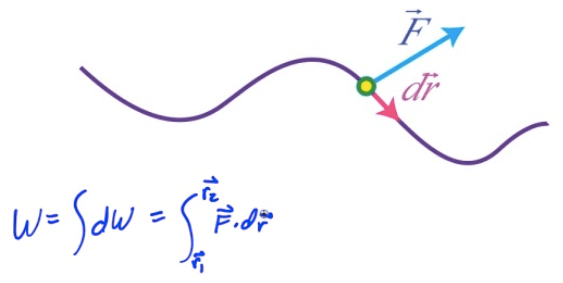# Work-Energy Theorem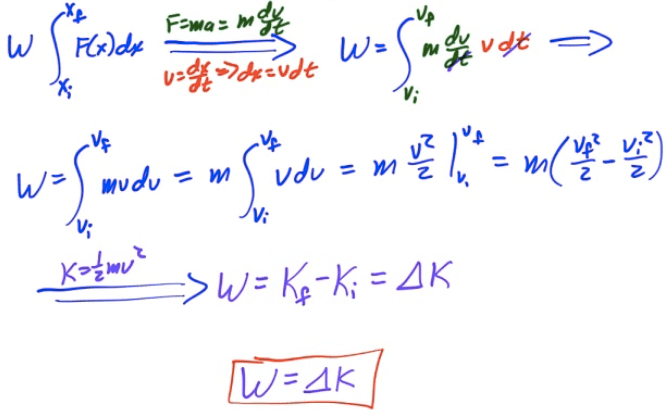# Example: Velocity from an F-d Graph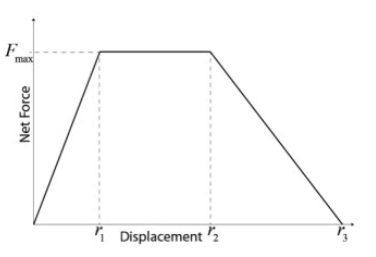• Determine the object's final speed.